Name:    Quiz 7.1-7.4

Multiple Choice
Identify the choice that best completes the statement or answers the question.

Find the volume.

1.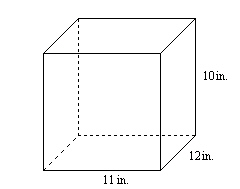a. 132 in.3 c. 480 in.3 b. 33 in.3 d. 1320 in.3

2.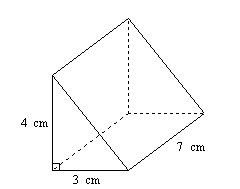a. 14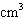c. 42b. 84d. 633.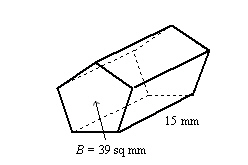a. 54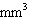c. 114b. 153d. 585Find the volume.

4.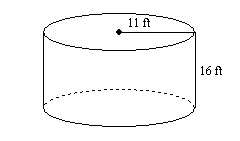a.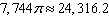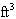c.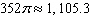b.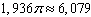d.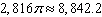5.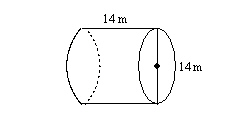a.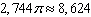m3 c.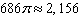m3 b.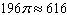m3 d.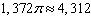m3

Find the volume.

6.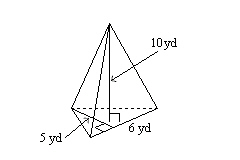a.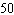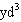c. 21b. 300d.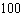7.

The volume of a cone is 19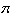cubic meters. What is the volume of a cylinder having the same base and same height?
 a.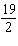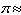29.8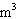c. 57179b.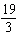19.9d. 57Numeric Response

1.

You pour 1 liters of water into an empty vase shaped like an octagonal prism. The base area is 50 square centimeters. What is the height of the water? (1 L = 1000 cm3)

2.

A cylinder has a surface area of 2826 square meters and a radius of 15 meters. Find the volume of the cylinder to the nearest whole number.

1.

Complete the table to find the area of the base B or the height h of the pyramid.

 Volume, V Area of Base, B Height, h a.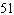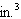9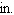b.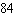36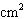c.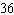36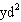2.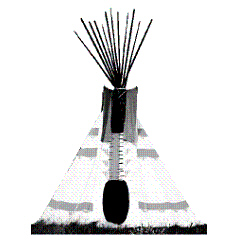a.   What is the shape of the base of the teepee?
b.   Your teepee’s height is about 10 feet. Estimate the volume of the teepee.